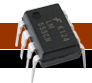# 555 Timer Circuits

555 Timers are fun and a great way to start learning electronics

## 555 Timer Calculator

This page is very useful when creating 555 timer circuits. it will allow you to calculate the frequency and duty cycle.

Astable 555 Square Wave Calculator

In an astable circuit, the output continually switches state between high and low without any intervention from the user. The duration of the high and low states are based on what values you choose for R1, R2 and C1.

The formulas used are:
Time High (secs) = 0.693 * (R1 + R2) * C1
Time Low (secs) = 0.693 * R2 * C1
Frequency = 1.44 / ((R1 + R2 + R2) * C1)
Duty Cycle Percentage = (Th / (Th + Tl)) * 100

 Resistor (R1) Ohms Resistor (R2) Ohms Capacitor (C1) Farads Enter R1, R2 and C1, then press Calculate

Monostable 555 Timeout Calculator

This calculator is designed to give the timeout value (D) for a Monostable 555 Timer Circuit. When power is applied to a monostable circuit the output is low until the delay time has elapsed, the output then goes high and remains high.

The formula used is:
Time Out Delay (secs) = 1.1 * R1 * C1

To use this calculator simply enter values for R1 and C1, then press Calculate.

 Resistor (R1) Ohms Capacitor (C1) Farads Enter R1 and C1, then press Calculate

Next Page:
Common Mistakes
Prev Page:
Using the Output of a 555 Timer